Courses

# Chapter 6 - Root Locus - Notes, Control System, Electrical Engineering Electrical Engineering (EE) Notes | EduRev

## Electrical Engineering (EE) : Chapter 6 - Root Locus - Notes, Control System, Electrical Engineering Electrical Engineering (EE) Notes | EduRev

The document Chapter 6 - Root Locus - Notes, Control System, Electrical Engineering Electrical Engineering (EE) Notes | EduRev is a part of the Electrical Engineering (EE) Course Electrical Engineering SSC JE (Technical).
All you need of Electrical Engineering (EE) at this link: Electrical Engineering (EE)

## ROOT LOCUS

• The Routh's criterion gives a satisfactory answer to the question of stability but its adoption to determine the relative stability is not satisfactory and requires trial and error procedure even in the analysis problem.
• A simple technique, known as the root locus technique, for finding the roots of the characteristic equation, introduced by W.R.Evens, is extensively used in control engineering practice.
• This technique provides a graphical method of plotting the locus of the roots in the s-plane as a given system parameter is varied over the complete range of values (may be from zero to infinity).
• The roots corresponding to a particular value of the system parameter can then be located on the locus or the value of the parameter for a desired root location can be determined from the locus.
• Root locus is drawn with the help of spirule

• The roots locus is a powerful technique as it brings into focus the complete dynamic response of the system and further, being a graphical technique, an approximate root locus sketch can be made quickly and the designer can easily visualize the effects of varying various system parameters on root locations.
• The root locus also provides a measure of sensitivity of roots to the variation in the parameter being considered.
• The root locus also provides a measure of sensitivity of roots to the variation in the parameter being considered.
• It may further be pointed out here that the root locus technique is applicable for single as well as multiple-loop system.
• In short it is defined as the locus of the roots of the characteristic equation as the gain parameter 'K' varies from 0 to ∞

ANGLE & MAGNITUDE CONDITIONS
Angle condition

• The angle condition is used for checking whether particular points are lying on root locus or not

1 + G(s)H(s) = 0

G(s)H(s) = -1

G(s)H(s) = -1 +10

∠G(s)H(s) = 1800

∠G(s)H(s) = +- (2q + 1)1800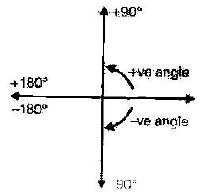• The angle condition may be stated as for a point to lie on root locus, the angle evaluated at that point must be an odd multiple of ±180º . Magnitude Condition
• This condition is used for finding the value of system gain K at that point on root locus.

G(s)H(s) =1

RULES OF DRAWING THE ROOT LOCUS

• Root locus starts from open loop poles with K= 0 (although practically it never happens as practically we have number of poles greater then number of zeros); and ends on open loop zeros with K = ∞
• Root locus is always symmetrical about real axis.
• A point on real axis lies on the root locus if number of poles + zeros to the right of the point are odd.

Steps of Drawing the Root Locus
Let, Number of poles = n (open loop poles)
Number of open loop zeros = m

• Number of root loci ending on infinite = n - m, n > m
• Root locus on real axis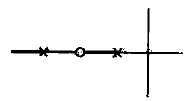• Here the root locus on real axis confirms above mentioned rule.
• Root locus moves always away from open loop poles and towards zero or infinity.
•  Number of asymptotes = (n – m)
•  Asymptotes are the paths along which root locus moves towards ∞ .
•  Angle of asymptotes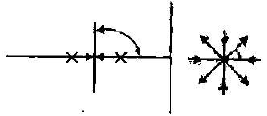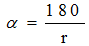r = Number of incoming branch of root locus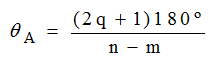q = 0, 1, 2, ......, n – m – 1

(e) Centroid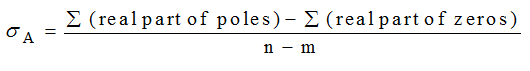(f) Determination of Breakaway or breaking point put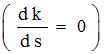and find out the value of 's'.

(g) Angle of departure or Angle of arrival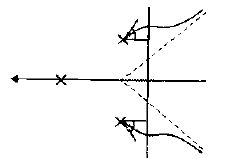• Angle made by root locus with real axis when it departs from a complex open loop pole is called angle of departure.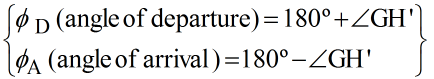∠GH' = angle of the function excluding the concerned poles at the poles itself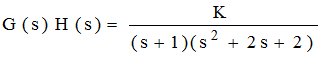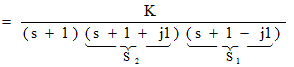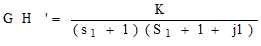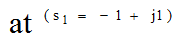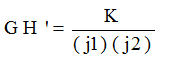• Just calculate for one (s1, or s2) and you can write for the other by putting negative sign.
• Crossover at imaginary axis.
• The roots of the auxiliary equation in Routh array at K = Kmar determines the intersection of rootlocus with imaginary axis.
• Determination of 'K' from root-locus: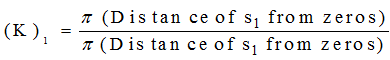i.e for the following root locus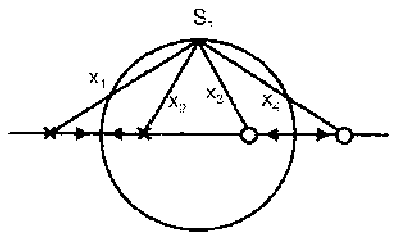Table: Open-loop pole-zero configurations and the corresponding Root loci.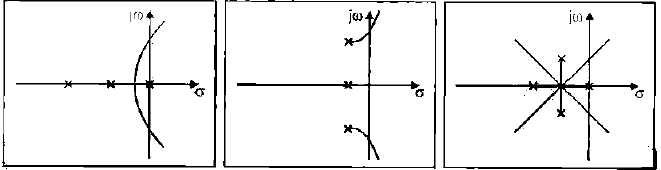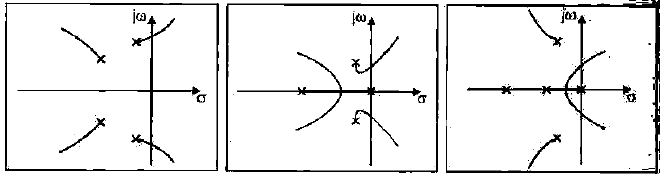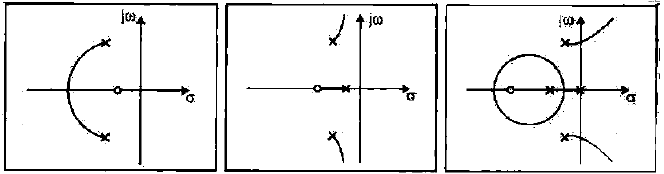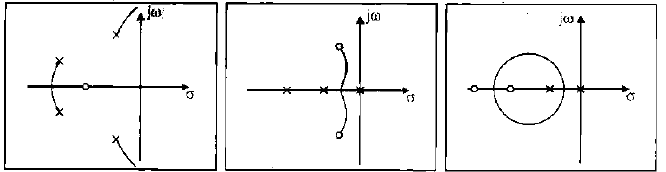Offer running on EduRev: Apply code STAYHOME200 to get INR 200 off on our premium plan EduRev Infinity!

65 docs|37 tests

,

,

,

,

,

,

,

,

,

,

,

,

,

,

,

,

,

,

,

,

,

,

,

,

,

,

,

;